Ex 4.1

Chapter 4 Class 11 Mathematical Induction
Serial order wise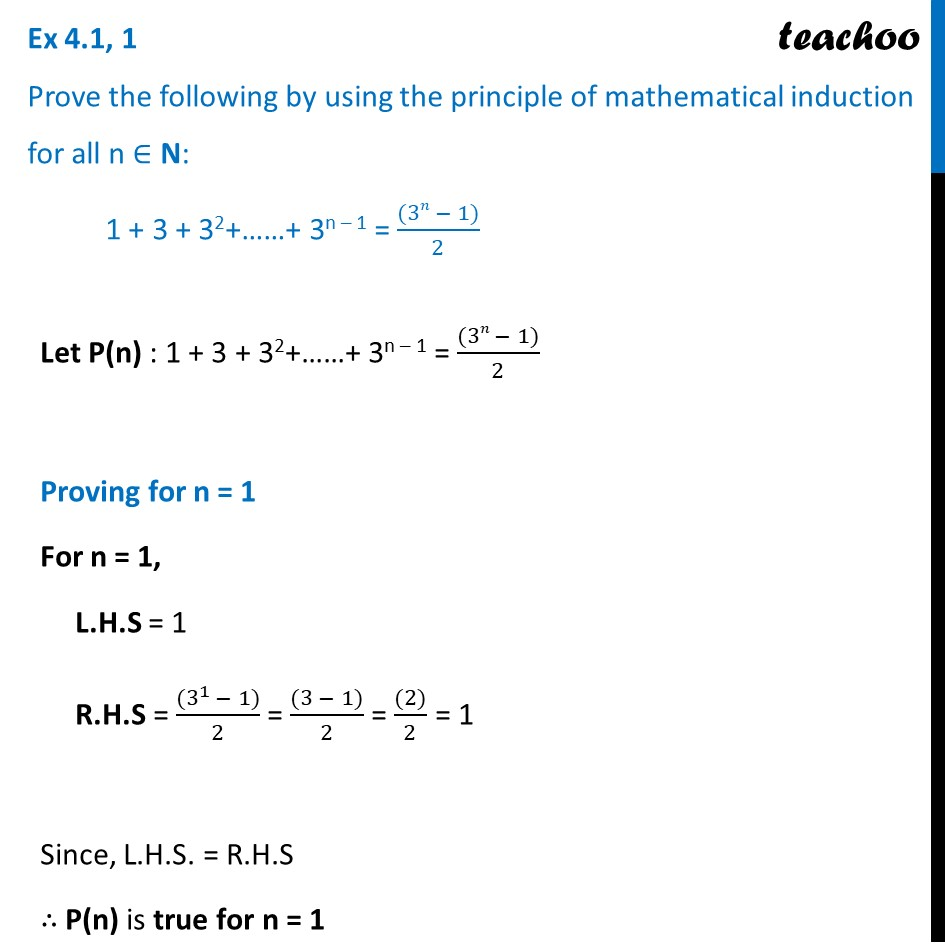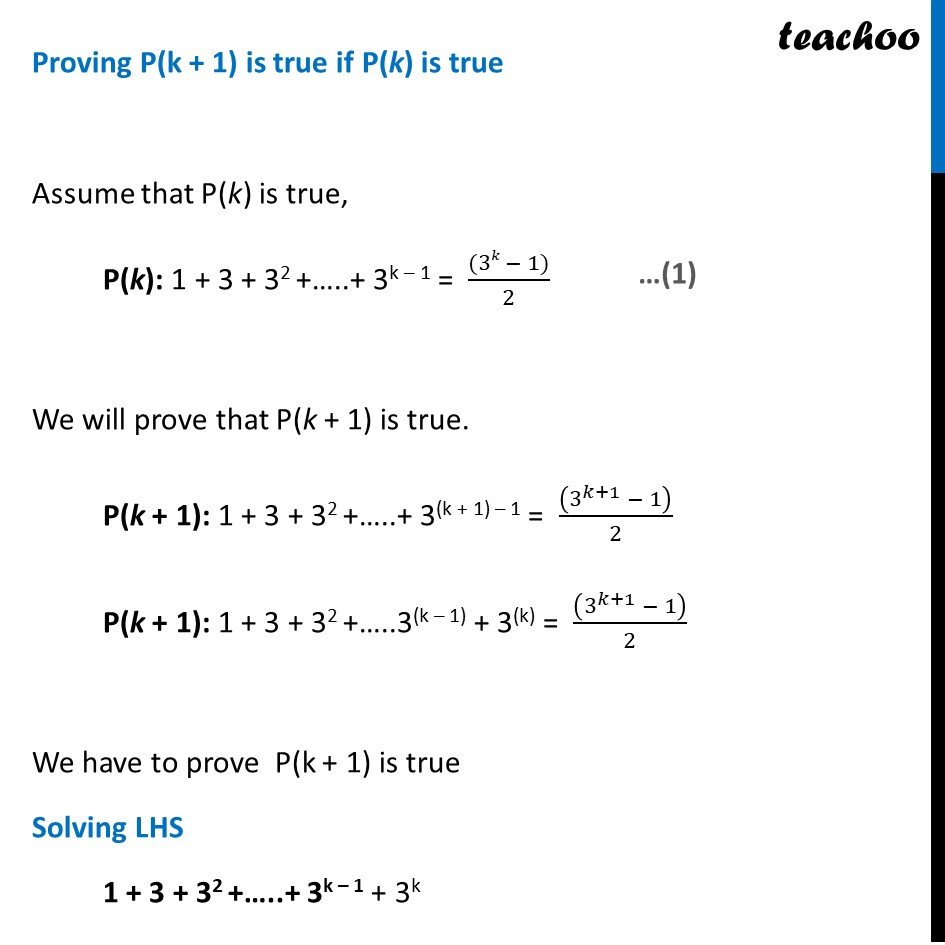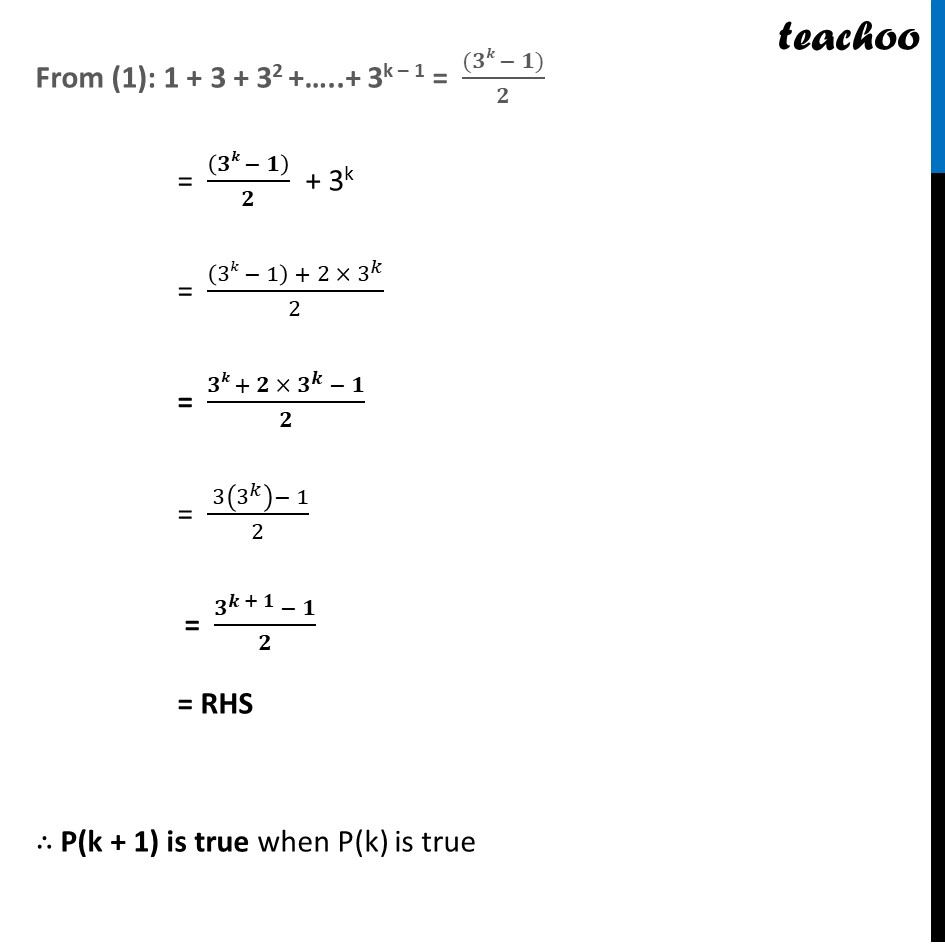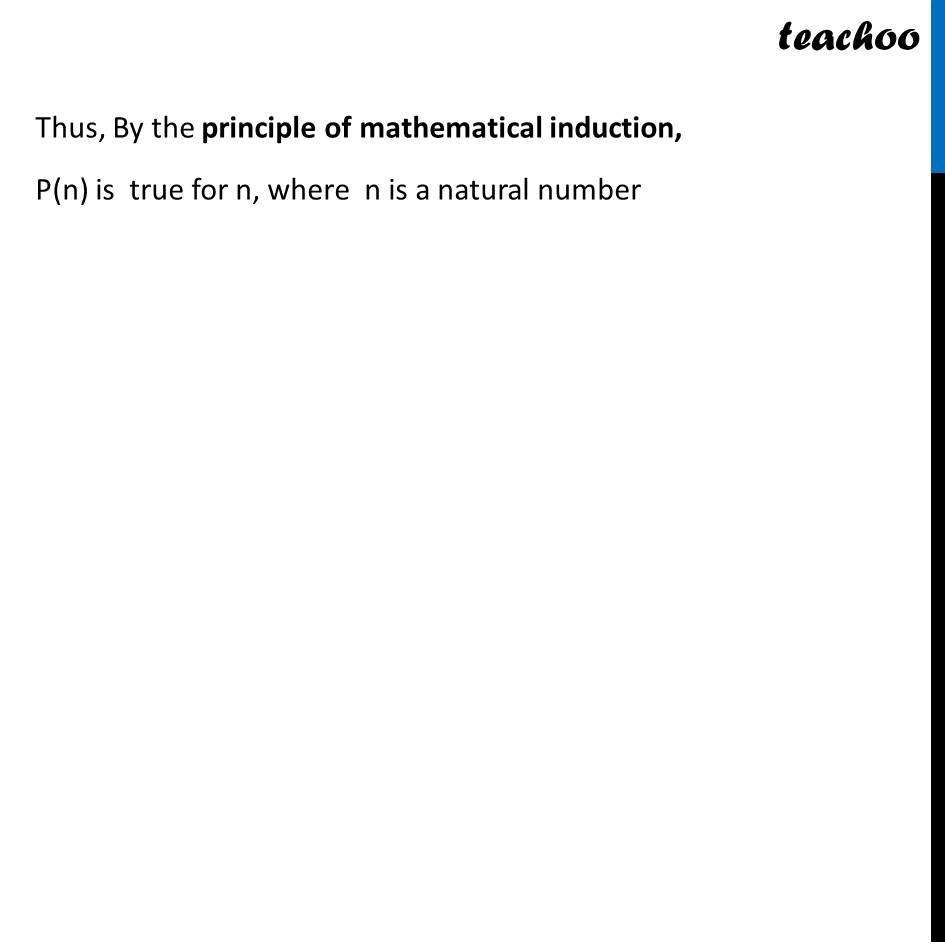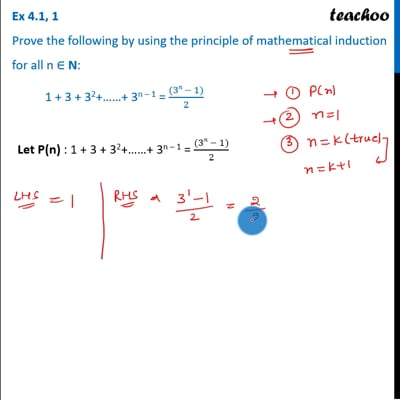This video is only available for Teachoo black users

Introducing your new favourite teacher - Teachoo Black, at only ₹83 per month

### Transcript

Ex 4.1, 1 Prove the following by using the principle of mathematical induction for all n ∈ N: 1 + 3 + 32+……+ 3n – 1 = ((3𝑛 − 1))/2 Let P(n) : 1 + 3 + 32+……+ 3n – 1 = ((3𝑛 − 1))/2 Proving for n = 1 For n = 1, L.H.S = 1 R.H.S = ((3^1 − 1))/2 = ((3 − 1))/2 = ((2))/2 = 1 Since, L.H.S. = R.H.S ∴ P(n) is true for n = 1 Proving P(k + 1) is true if P(k) is true Assume that P(k) is true, P(k): 1 + 3 + 32 +…..+ 3k – 1 = ((3𝑘 − 1))/2 We will prove that P(k + 1) is true. P(k + 1): 1 + 3 + 32 +…..+ 3(k + 1) – 1 = ((3^(𝑘+1) − 1))/2 P(k + 1): 1 + 3 + 32 +…..3(k – 1) + 3(k) = ((3^(𝑘+1) − 1))/2 We have to prove P(k + 1) is true Solving LHS 1 + 3 + 32 +…..+ 3k – 1 + 3k From (1): 1 + 3 + 32 +…..+ 3k – 1 = ((𝟑𝒌 − 𝟏))/𝟐 = ((𝟑𝒌 − 𝟏))/𝟐 + 3k = ((3𝑘 − 1) + 2 × 3^𝑘)/2 = (𝟑𝒌 + 𝟐 × 𝟑^𝒌 − 𝟏)/𝟐 = ( 3(3^𝑘 )− 1)/2 = (𝟑^(𝒌 + 𝟏) − 𝟏)/𝟐 = RHS ∴ P(k + 1) is true when P(k) is true Thus, By the principle of mathematical induction, P(n) is true for n, where n is a natural number